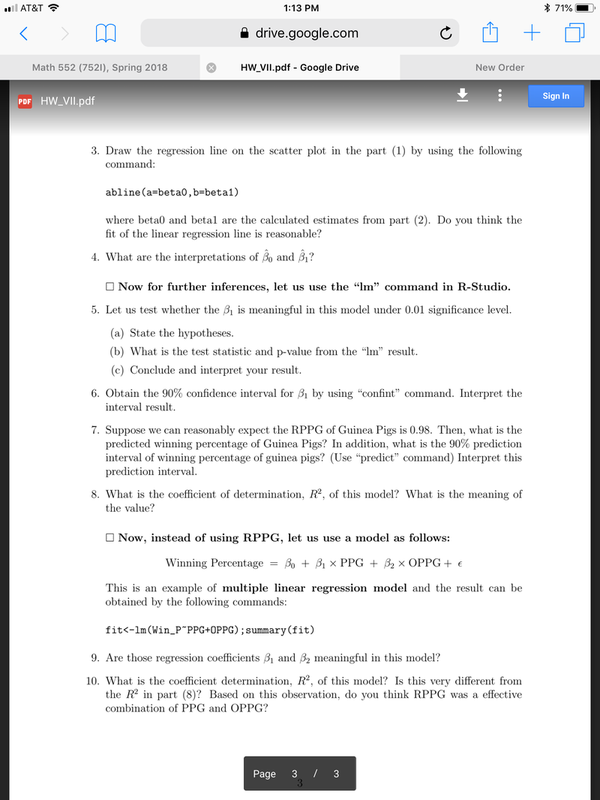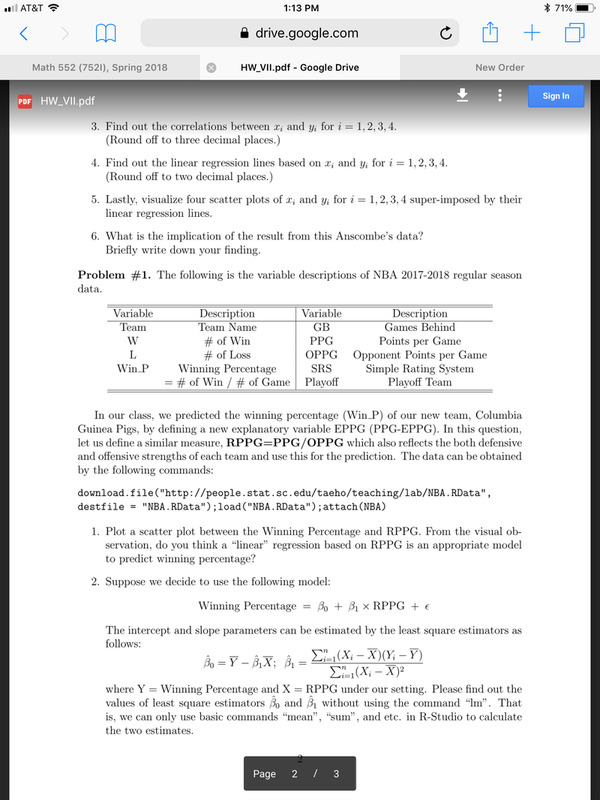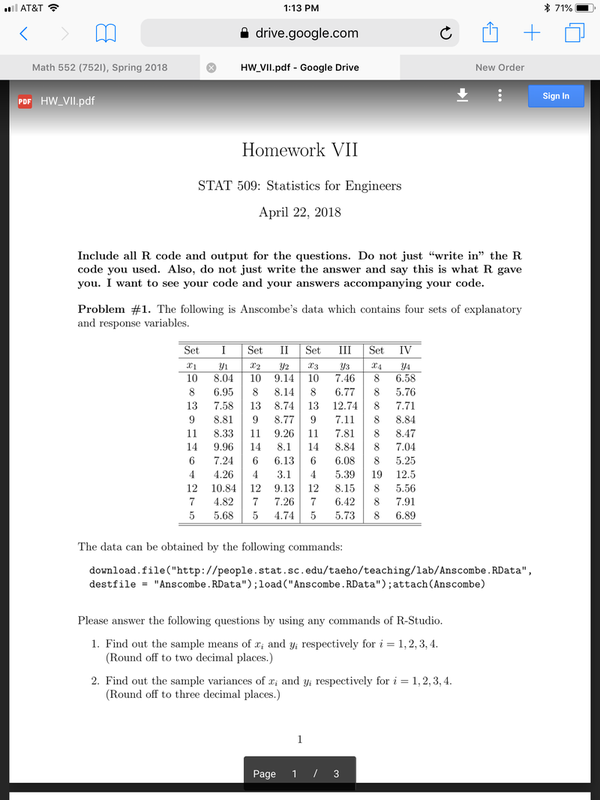# We Helped With This Statistics Homework: Have A Similar One?

SOLVEDCategory Math Statistics College Solved Assignment Statistics

## Short Assignment Requirements

Statistics homework for r studio. Questions submitted below in photos

## Assignment ImageAT&T < Math 552 (7521), Spring 2018 PDF HW_VII.pdf abline (a=beta0,b=beta1) drive.google.com 1:13 PM HW_VII.pdf - Google Drive 3. Draw the regression line on the scatter plot in the part (1) by using the following command: where beta0 and betal are the calculated estimates from part (2). Do you think the fit of the linear regression line is reasonable? 4. What are the interpretations of Bo and B₁? Now for further inferences, let us use the "lm" command in R-Studio. 5. Let us test whether the B₁ is meaningful in this model under 0.01 significance level. (a) State the hypotheses. (b) What is the test statistic and p-value from the "lm" result. (c) Conclude and interpret your result. 6. Obtain the 90% confidence interval for ₁ by using "confint" command. Interpret the interval result. 7. Suppose we can reasonably expect the RPPG of Guinea Pigs is 0.98. Then, what is the predicted winning percentage of Guinea Pigs? In addition, what is the 90% prediction interval of winning percentage of guinea pigs? (Use "predict" command) Interpret this prediction interval. New Order Now, instead of using RPPG, let us use a model as follows: Bo + ₁ x PPG + ₂ × OPPG+ € 8. What is the coefficient of determination, R²2, of this model? What is the meaning of the value? = Page Winning Percentage This is an example of multiple linear regression model and the result can be obtained by the following commands: fit<-lm (Win_P~PPG+OPPG) ; summary (fit) 9. Are those regression coefficients ₁ and 3₂ meaningful in this model? 10. What is the coefficient determination, R², of this model? Is this very different from the R² in part (8)? Based on this observation, do you think RPPG was a effective combination of PPG and OPPG? + / 3 3 3 71% Sign In

## Assignment ImageAT&T < Math 552 (7521), Spring 2018 PDF HW_VII.pdf 1:13 PM drive.google.com 3. Find out the correlations between x; and y; for i = 1, 2, 3, 4. (Round off to three decimal places.) HW_VII.pdf - Google Drive 4. Find out the linear regression lines based on xi and Yi for i=1, 2, 3, 4. (Round off to two decimal places.) 5. Lastly, visualize four scatter plots of Xi and yi linear regression lines. Variable Team W L Win P 6. What is the implication of the result from this Anscombe's data? Briefly write down your finding. Description Team Name # of Win # of Loss Problem #1. The following is the variable descriptions of NBA 2017-2018 regular season data. Winning Percentage = # of Win / # of Game for i Variable GB PPG OPPG SRS Playoff Bo = Y – BLX; Bị In our class, we predicted the winning percentage (Win_P) of our new team, Columbia Guinea Pigs, by defining a new explanatory variable EPPG (PPG-EPPG). In this question, let us define a similar measure, RPPG=PPG/OPPG which also reflects the both defensive and offensive strengths of each team and use this for the prediction. The data can be obtained by the following commands: 1, 2, 3, 4 super-imposed by their download.file("http://people.stat.sc.edu/taeho/teaching/lab/NBA.RData", destfile = "NBA.RData"); load ("NBA. RData"); attach (NBA) = = 1. Plot a scatter plot between the Winning Percentage and RPPG. From the visual ob- servation, do you think a “linear” regression based on RPPG is an appropriate model to predict winning percentage? 2. Suppose we decide to use the following model: Winning Percentage = New Order Description Games Behind Points per Game Opponent Points per Game Simple Rating System Playoff Team The intercept and slope parameters can be estimated by the least square estimators as follows: Bo + ₁ x RPPG + € Σ₁ (Xi - X)(Yi – Y) Σ₁₁(X₂ – X)² Page 2 / 3 where Y Winning Percentage and X RPPG under our setting. Please find out the values of least square estimators Bo and ₁ without using the command "Im". That is, we can only use basic commands "mean", "sum", and etc. in R-Studio to calculate the two estimates. + 71% Sign In

## Assignment ImageIs it free to get my assignment evaluated?

Yes. No hidden fees. You pay for the solution only, and all the explanations about how to run it are included in the price. It takes up to 24 hours to get a quote from an expert. In some cases, we can help you faster if an expert is available, but you should always order in advance to avoid the risks. You can place a new order here.

How much does it cost?

The cost depends on many factors: how far away the deadline is, how hard/big the task is, if it is code only or a report, etc. We try to give rough estimates here, but it is just for orientation (in USD):

 Regular homework \$20 - \$150 Advanced homework \$100 - \$300 Group project or a report \$200 - \$500 Mid-term or final project \$200 - \$800 Live exam help \$100 - \$300 Full thesis \$1000 - \$3000

How do I pay?

Credit card or PayPal. You don't need to create/have a Payal account in order to pay by a credit card. Paypal offers you "buyer's protection" in case of any issues.

Why do I need to pay in advance?

We have no way to request money after we send you the solution. PayPal works as a middleman, which protects you in case of any disputes, so you should feel safe paying using PayPal.

Do you do essays?

No, unless it is a data analysis essay or report. This is because essays are very personal and it is easy to see when they are written by another person. This is not the case with math and programming.

Why there are no discounts?

It is because we don't want to lie - in such services no discount can be set in advance because we set the price knowing that there is a discount. For example, if we wanted to ask for \$100, we could tell that the price is \$200 and because you are special, we can do a 50% discount. It is the way all scam websites operate. We set honest prices instead, so there is no need for fake discounts.

Do you do live tutoring?

No, it is simply not how we operate. How often do you meet a great programmer who is also a great speaker? Rarely. It is why we encourage our experts to write down explanations instead of having a live call. It is often enough to get you started - analyzing and running the solutions is a big part of learning.

What happens if I am not satisfied with the solution?

Another expert will review the task, and if your claim is reasonable - we refund the payment and often block the freelancer from our platform. Because we are so harsh with our experts - the ones working with us are very trustworthy to deliver high-quality assignment solutions on time.

Customer Feedback

"Thanks for explanations after the assignment was already completed... Emily is such a nice tutor! "

Order #13073